# Olympiad Test: Direction Sense Test - Class 5

Test Description

## 20 Questions MCQ Test National Cyber Olympiad Class 5 - Olympiad Test: Direction Sense Test

Olympiad Test: Direction Sense Test for Class 5 2023 is part of National Cyber Olympiad Class 5 preparation. The Olympiad Test: Direction Sense Test questions and answers have been prepared according to the Class 5 exam syllabus.The Olympiad Test: Direction Sense Test MCQs are made for Class 5 2023 Exam. Find important definitions, questions, notes, meanings, examples, exercises, MCQs and online tests for Olympiad Test: Direction Sense Test below.
 1 Crore+ students have signed up on EduRev. Have you?
Olympiad Test: Direction Sense Test - Question 1

### Direction: Dev, Kumar, Nilesh, Ankur and Pintu are standing facing North in a playground such as given below: • Kumar is at 40 m to the right of Ankur. • Dev is 60 m to the south of Kumar. • Nilesh is at a distance of 25 m to the west of Ankur. • Pintu is at a distance of 90 m to the North of Dev. Q. Who is in the North-East direction of the person who is to the left of Kumar?

Detailed Solution for Olympiad Test: Direction Sense Test - Question 1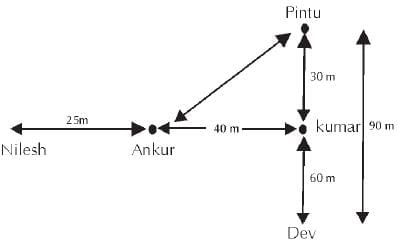Ankur is towards the left of Kumar.
Hence Pintu is in North-East of Ankur.

Olympiad Test: Direction Sense Test - Question 2

### Direction: Dev, Kumar, Nilesh, Ankur and Pintu are standing facing North in a playground such as given below: • Kumar is at 40 m to the right of Ankur. • Dev is 60 m to the south of Kumar. • Nilesh is at a distance of 25 m to the west of Ankur. • Pintu is at a distance of 90 m to the North of Dev. Q. If a boy started walking from Nilesh’s position, met Ankur and then Kumar and Dev and then to Pintu. How much total distance did he cover while walking from one person to another?

Detailed Solution for Olympiad Test: Direction Sense Test - Question 2

Required distance = 25 m + 40 m + 60 m + 90 m Required distance = 215 m

Olympiad Test: Direction Sense Test - Question 3

### Direction: Each of the following questions is based on the following information. • Six flats on a floor in two rows facing North and South are allotted to P, Q, R, S, T and U. • Q gets a North facing flat and is not next to S. • S and U get diagonally opposite flats. • R next to U, gets a south facing flat and T gets North facing flat. Q. If the flats of P and T are interchanged, then who’s flat will be next to that of U?

Detailed Solution for Olympiad Test: Direction Sense Test - Question 3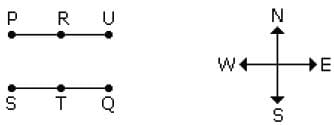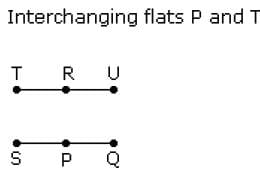Olympiad Test: Direction Sense Test - Question 4

Direction: Each of the following questions is based on the following information.
• Six flats on a floor in two rows facing North and South are allotted to P, Q, R, S, T and U.
• Q gets a North facing flat and is not next to S.
• S and U get diagonally opposite flats.
• R next to U, gets a south facing flat and T gets North facing flat.

Q. Which of the following combination gets the south-facing flats?

Detailed Solution for Olympiad Test: Direction Sense Test - Question 4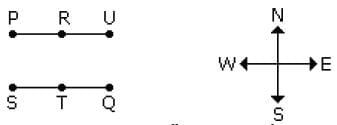Hence URP flat combination gets south-facing flats.

Olympiad Test: Direction Sense Test - Question 5

Direction: Each of the following questions is based on the following information.
• Six flats on a floor in two rows facing North and South are allotted to P, Q, R, S, T and U.
• Q gets a North facing flat and is not next to S.
• S and U get diagonally opposite flats.
• R next to U, gets a south facing flat and T gets North facing flat.

Q. The flats of which of the pair other than SU, is diagonally opposite to each other?

Detailed Solution for Olympiad Test: Direction Sense Test - Question 5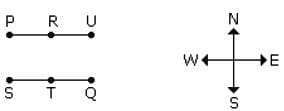Hence, Q and P are diagonally opposite to each other.

Olympiad Test: Direction Sense Test - Question 6

Direction: Each of the following questions is based on the following information.
• Six flats on a floor in two rows facing North and South are allotted to P, Q, R, S, T and U.
• Q gets a North facing flat and is not next to S.
• S and U get diagonally opposite flats.
• R next to U, gets a south facing flat and T gets North facing flat.

Q. Whose flat is between Q and S?

Detailed Solution for Olympiad Test: Direction Sense Test - Question 6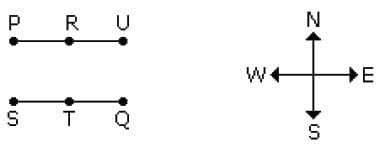Hence flat T is between Q and S.

Olympiad Test: Direction Sense Test - Question 7

Direction: Each of the following questions is based on the following information.
• 8-trees → mango, guava, papaya, pomegranate, lemon, banana, raspberry and apple are in two rows 4 in each facing North and South.
• Lemon is between mango and apple but opposite to guava.
• Banana is at one end of the line and is next to the right of guava or either banana tree is  after guava tree.
• Raspberry tree which at one end of a line, is diagonally opposite to mango tree.

Q. Which of the following statements is definitely true?

Detailed Solution for Olympiad Test: Direction Sense Test - Question 7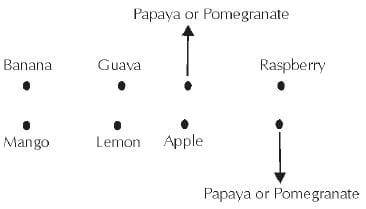Olympiad Test: Direction Sense Test - Question 8

Direction: Each of the following questions is based on the following information.
• 8-trees → mango, guava, papaya, pomegranate, lemon, banana, raspberry and apple are in two rows 4 in each facing North and South.
• Lemon is between mango and apple but opposite to guava.
• Banana is at one end of the line and is next to the right of guava or either banana tree is  after guava tree.
• Raspberry tree which at one end of a line, is diagonally opposite to mango tree.

Q. Which tree is opposite to raspberry tree?

Detailed Solution for Olympiad Test: Direction Sense Test - Question 8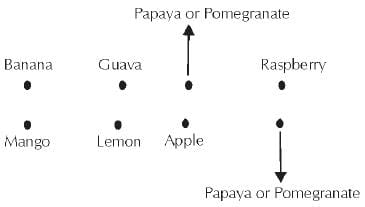Olympiad Test: Direction Sense Test - Question 9

Direction: Each of the following questions is based on the following information.
• 8-trees → mango, guava, papaya, pomegranate, lemon, banana, raspberry and apple are in two rows 4 in each facing North and South.
• Lemon is between mango and apple but opposite to guava.
• Banana is at one end of the line and is next to the right of guava or either banana tree is  after guava tree.
• Raspberry tree which at one end of a line, is diagonally opposite to mango tree.

Q. Which tree is opposite to the banana tree?

Detailed Solution for Olympiad Test: Direction Sense Test - Question 9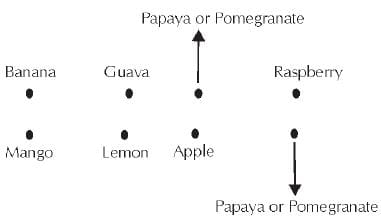Olympiad Test: Direction Sense Test - Question 10

Direction: Each of the following questions is based on the following information.
• A # B means B is at 1 metre to the right of A.
• A \$ B means B is at 1 metre to the North of A.
• A * B means B is at 1 metre to the left of A.
• A @ B means B is at 1 metre to the south of A.
• In each question, first person from the left is facing North.

Q. According to X @ B * P, P is in which direction with respect to X?

Detailed Solution for Olympiad Test: Direction Sense Test - Question 10

According to X @ B * P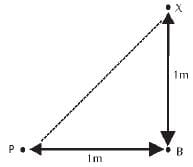Hence, P is in South-West of X.

Olympiad Test: Direction Sense Test - Question 11

Direction: Each of the following questions is based on the following information.
• A # B means B is at 1 metre to the right of A.
• A \$ B means B is at 1 metre to the North of A.
• A * B means B is at 1 metre to the left of A.
• A @ B means B is at 1 metre to the south of A.
• In each question, first person from the left is facing North.

Q. According to M # N \$ T, T is in which direction with respect to M?

Detailed Solution for Olympiad Test: Direction Sense Test - Question 11

According to M # N \$ T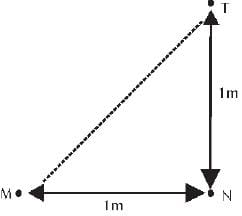Hence T is in the North-East of M.

Olympiad Test: Direction Sense Test - Question 12

Direction: Each of the following questions is based on the following information.
• A # B means B is at 1 metre to the right of A.
• A \$ B means B is at 1 metre to the North of A.
• A * B means B is at 1 metre to the left of A.
• A @ B means B is at 1 metre to the south of A.
• In each question, first person from the left is facing North.

Q. According to P # R \$ A * U, in which direction is U with respect to P?

Detailed Solution for Olympiad Test: Direction Sense Test - Question 12

According to P # R \$ A * U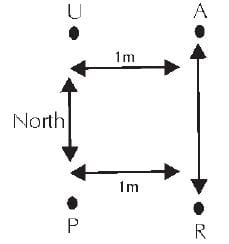Hence U is in North direction with respect to P.

Olympiad Test: Direction Sense Test - Question 13

Direction: Find the correct answer for the following question based on related details given.

Q. Golu starts from his house towards West. After walking a distance of 30 m, he turned towards right and walked 20 m ahead. He then turned left and moving a distance of 10 m, turned to his left again and walked 40 m. He then turned to the left and walked 5 m. Finally he turned to his left. In which direction is he walking now?

Olympiad Test: Direction Sense Test - Question 14

Direction: Find the correct answer for the following question based on related details given.

Q. A rat runs towards East and turns right twice, runs and then turns to left, runs and then turns left, runs and finally turns left and runs. Now, in which direction is the rat running now?

Olympiad Test: Direction Sense Test - Question 15

Direction: Find the correct answer for the following question based on related details given.

Q. Suraj walks 10 m towards South. Turning to the left, he walks 20 m and then turns right. After moving a distance of 20 m, he turns right and walks another 20 m. Finally, he turns right and moves a distance of 10 m. How far and in which direction is he from the starting point?

Olympiad Test: Direction Sense Test - Question 16

Direction: Find the correct answer for the following question based on related details given.

Q. I am facing South. I turn right and walk 20 m. Then I turn right again and walk 10 m. Then I turn left and walk 10 m and then turning right walk 20 m. Then I turn right again and walk 60 m. In which direction am I from the starting point?

Olympiad Test: Direction Sense Test - Question 17

Direction: Find the correct answer for the following question based on related details given.

Q. Anil went 15  Km towards west from my house, then turned left and walked 20  Km. He then turned East and walked 25  Km and finally turning left covered 20  Km. How far was he from his house?

Olympiad Test: Direction Sense Test - Question 18

Direction: Find the correct answer for the following question based on related details given.

Q. Rashmi walks 20 m North. Then she turns right and walks 30 m. Then she turns right and walks 35 m. Then she turns left and walks 15 m. Then she again turns left and walks 15 m. In which direction and how many metres away is she from her original position?

Olympiad Test: Direction Sense Test - Question 19

Direction: Find the correct answer for the following question based on related details given.

Q. The door of Adi’s house faces East. From the back side of his house, he walks straight 50 metres, then turns to the right and walks 50 metres again. Finally, he turns towards left and stops after walking 25 m. Now, Adi is in which direction from the starting point?

Olympiad Test: Direction Sense Test - Question 20

Direction: Find the correct answer for the following question based on related details given.

Q. If A is to the south of B and C is to the east of B, in what direction is A with respect to C?

## National Cyber Olympiad Class 5

1 videos|22 tests(Scan QR code)